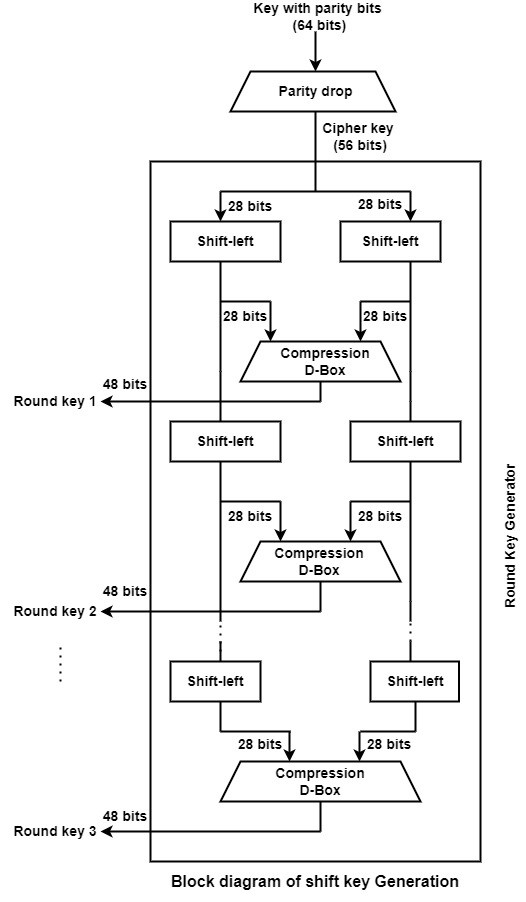# What are the following steps for the key generation of DES in information security?

Data Encryption Standard (DES) is a block cipher algorithm that creates plain text in blocks of 64 bits and transform them to ciphertext using keys of 48 bits. It is a symmetric key algorithm, which defines that the similar key is used for encrypting and decrypting information.

DES takes a 64-bit plaintext and produce a 64-bit ciphertext; at the decryption site, DES takes a 64-bit ciphertext and produce a 64-bit block of plaintext. The same 56-bit cipher key can be used for both encryption and decryption.

The key nature of DES is that the algorithms is fixed and is public data. However, the actual key used is shared secret among the originator and the receiver of a transmission.

Advances in DES contains lengthening a key to 128 bits and the multi-pass DES which includes multiple passes generally three of encryption and decryption using multiple keys.

DES was the outcome of a research project install by International Business Machines (IBM) Corporation in the late 1960’s which resulted in a cipher called a LUCIFER. In the initial 1970’s it was determined to degrade LUCIFER and a multiple innovation were introduced.

The algorithm implement 16 rounds of encryption and for each round, a unique key is produced. Before transforming to the steps, it is essential to understand that in plaintext the bits are labeled from 1 to 64 where 1 is the most significant bit and 64 is the least significant bit. The process of generating keys are as follows −

• The round key generator produce sixteen 48-bit keys out of a 56-bit cipher key. The cipher key is provided as 64 bit key in which 8 extra bits are parity bits, which are discarded before the actual key generation process begins.

• The parity bit drop process drops the parity bits (bit 8, 16, 24, 32…64) from the 64-bit key and permutes the remaining bit according to the pre-defined rules as display in the parity bit drop table below.

• These remaining 56 bits are generally used for key generation.

• After the permutation, the keys are divided into two 28 bits parts. Each part is changed left one or two bits is depend on the rounds.

• In round 1, 2, 9, and 16 shifting is one bit and in the other rounds it is two bits. The two parts are integrate to build a 56 bit part.

• Thus the compression D-box transform it into 48 bit. These 48 bits are being utilized as a key for a round.

 57 49 41 33 25 17 09 01 58 50 42 34 26 18 10 02 59 51 43 35 27 19 11 03 60 52 44 36 63 55 47 39 31 23 15 07 62 54 46 38 30 22 14 06 61 53 45 37 29 21 13 05 28 20 12 04